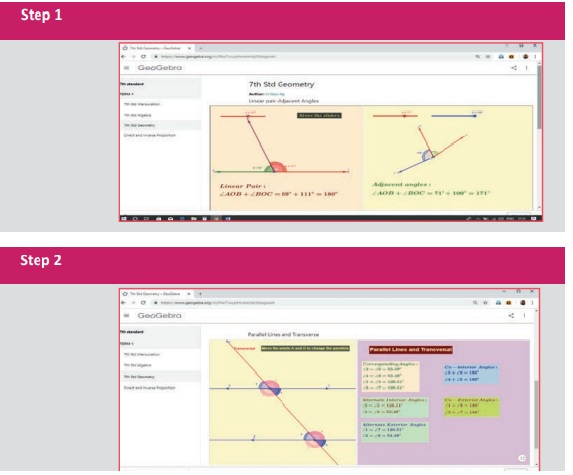Home | | Maths 7th Std | Summary

# Summary

7th Maths : Term 1 Unit 5 : Geometry : Summary

Summary

● Two angles which have a common vertex and a common arm, whose interiors do not overlap are called adjacent angles.

● The adjacent angles that are supplementary are called linear pair of angles.

● The sum of all the angles formed at a point on a straight line is 180°.

● The sum of the angles at a point is 360°.

● When two lines intersect each other, two pairs of non-adjacent angle formed are called vertically opposite angles.

● A transversal is a line that intersects two or more lines at distinct points.

● when two parallel lines are cut by a transversal,

◊ each pair of corresponding angles are equal.

◊ each pair of alternate Interior angles are equal.

◊ each pair of alternate exterior angles are equal.

◊ interior angles on the same side of the transversal are supplementary.

◊ exterior angles on the same side of the transversal are supplementary.

ICT Corner

Expected Result is shown in this pictureStep – 1 : Open the Browser type the URL Link given below (or) Scan the QR Code. GeoGebra work sheet named “7th std Geometry” will open. There are two activities 1. Linear pair- Adjacent Angles, 2. Parallel lines and Transverse.

In the second activity “Parallel lines and Transverse” move the points A and B and observe the different angles formed by the transverse line.

Step - 2 : Move the sliders to get, Parallelogram or Rectangle or Square and see the points given and observe.